Ch 6. Entropy Multimedia Engineering Thermodynamics Entropy Tds Relations EntropyChange IsentropicProcess IsentropicEfficiency EntropyBalance (1) EntropyBalance (2) ReversibleWork
 Chapter 1. Basics 2. Pure Substances 3. First Law 4. Energy Analysis 5. Second Law 6. Entropy 7. Exergy Analysis 8. Gas Power Cyc 9. Brayton Cycle 10. Rankine Cycle Appendix Basic Math Units Thermo Tables Search eBooks Dynamics Fluids Math Mechanics Statics Thermodynamics Author(s): Meirong Huang Kurt Gramoll ©Kurt GramollTHERMODYNAMICS - CASE STUDY SOLUTION A small power plant using hydraulic turbines is planned. The number of intake pipes which intake water from a reservoir to the turbines needs to be determined. Assumptions: Model the water as incompressible substance with a density ρ = 1000 kg/m3. Assume water flows through the pipes and turbines steadily, and the process is reversible.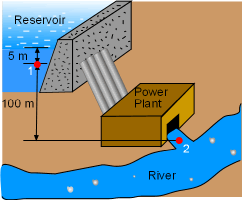Schematic of the Power Plant The schematic of the power plant is shown on the left. Take the pipes and the turbines together as a control volume. Denote the inlet of the intake pipes as 1 and the outflow of the turbine as 2.The reversible steady-flow work generated by this power plant is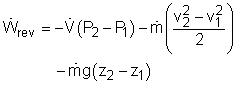where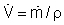The first term on the left-hand side is the pressure term. Point 1 is located 5 meter under the water surface in reservoir. So pressure P1 is       P1 = ρgh + Patm= 1000(9.8)(5) + 100,000            = 149,000 Pa = 1.49 bar Point 2 is located at the outflow of the power plant. Its pressure equals the ambient pressure,       P2 = 1.0 bar The second term on the left-hand side is the kinetic energy term. Water enters the pipes with a velocity of 1 m/s and leaves the turbine as the same velocity. Hence, there is no kinetic energy change when water flows from point 1 to point 2. That is,        ΔKE = 0 The third term on the left-hand side is the potential energy term. Water flowing from point 1 (100 m higher than point 2) to point 2 experiences a potential energy change of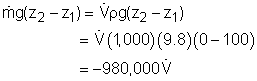Substituting the three terms into the expression for reversible steady-flow work gives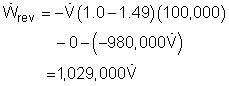This power plant needs to generate 1 MW power, hence,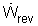= 1,000,000 W Substituting this to the expression of the reversible steady-flow work gives       1,000,000 = 1,029,000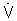= 0.97 m3/s Water enters the intake pipe with a velocity of 1 m/s. The flow rate through each 0.5 m diameter pipe is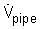= (3.14)(0.25)2(1) = 0.196 m3/s In order to intake 0.97 m3 of water through the pipes per second, the number of the pipes needed is       N =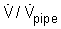= 0.97/0.196 = 5